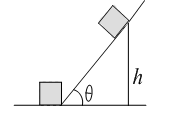# A cannon of mass 1000 kg located at the base of an inclined plane fires a shell of mass 50 kg in horizontal direction with velocity 180 km/h. The angle of inclination of the inclined plane with the horizontal is. The coefficient of friction between the cannon and inclined plane is 0.5. The maximum height, in metre, to which the cannon can ascend the inclined plane as a result of recoil is a)b)c)d) None of these

## Question ID - 100188 :- A cannon of mass 1000 kg located at the base of an inclined plane fires a shell of mass 50 kg in horizontal direction with velocity 180 km/h. The angle of inclination of the inclined plane with the horizontal is. The coefficient of friction between the cannon and inclined plane is 0.5. The maximum height, in metre, to which the cannon can ascend the inclined plane as a result of recoil is a)b)c)d) None of these

3537

(b)

Velocity of shell =180km/h=50m/s

Letbe the velocity of the cannot after firing, then from the conservation of linear momentum,Let the cannot go up by heightNet work done by external forcesWhereis the mass of cannon,

Solving, we getm

Next Question :
 A cylinder having moment of inertia, which isfree to rotate about its axis, receives an angular impulse ofinitially, followed by similar impulse after every 4 s. What is the angular speed of the cylinder 30 s after the initial impulse? a)b)c)d) Zero